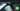# Hackerrank | Solution of Migratory Birds in Golang

October 22nd, 2018
|

In this one we will solve question migratory birds from hackerrank in golang. This one wraps a logic which can be used to solve many questions. Let's dive right into it.

# Problem Statement

The question can be found at this link. The problem statement states that there are many birds of very few types and we need to find which type of bird is most in number. In case we have multiple types with same number we need to answer the bird with lower type.

# Challenges

• Choose our preferred language as golang on hackerrank. The moment we do that, we get some 50-60 lines of code which are very unfamiliar to someone who is new to language.
• Cracking the logic required to solve the question.
• A keen interest in bird watching

# Solution

This is the template you get on hackerrank for this problem statement.

``````    package main

import (
"bufio"
"fmt"
"io"
"os"
"strconv"
"strings"
)

// Complete the migratoryBirds function below.
func migratoryBirds(arr []int32) int32 {

}

func main() {

stdout, err := os.Create(os.Getenv("OUTPUT_PATH"))
checkError(err)

defer stdout.Close()

writer := bufio.NewWriterSize(stdout, 16 * 1024 * 1024)

checkError(err)

var arr []int32

for i := 0; i < int(arrCount); i++ {
arrItemTemp, err := strconv.ParseInt(arrTemp[i], 10, 64)
checkError(err)
arrItem := int32(arrItemTemp)
arr = append(arr, arrItem)
}

result := migratoryBirds(arr)

fmt.Fprintf(writer, "%d\n", result)

writer.Flush()
}

if err == io.EOF {
return ""
}

return strings.TrimRight(string(str), "\r\n")
}

func checkError(err error) {
if err != nil {
panic(err)
}
}``````
• First go ahead and delete everything in the pre-existing template.
• Let's write down our main wrapper function.
``````package main
import "fmt"

func main(){

}``````

I'll break this question into four parts. I just love this question.

• Declaring the required variables
• Deciding on our array
• Taking our array as input and doing some operations on it
• Writing the logic of the program and printing the result

So, let's look into the variables. According to the input format we are given the length of birds `n`, and in next line we are given the types of birds we encounter. If you look at the constraints of the types, it is between `1 and 5` , i.e. the type of bird can only be between 1 to 5. The number of birds are huge but the type is limited. We are required to find the bird type with highest number. So we can take an array with size `5` ( because our bird type is between 1 to 5 ) and when even we encounter a type we will add a counter at that index of the array. Since array index starts at 0, we also need to decrease the type by 1. So we need an array declaration, and a temporary variable `t` to hold the input. Let's go ahead and convert everything we discussed now into code.

``````	...
var n,t int

fmt.Scan(&n)

a := make([]int,5)

for i:=0;i<n;i++{
fmt.Scan(&t)
t = t -1
a[t]++
}``````

We are declaring the variables as discussed, creating an array, taking input for the array. Then we decrease the type by one to adjust for zero based indexing and increase the count for that index. Now we have count for all types of birds. Next we need our logic. So we need to display the type of bird whose occurrence was maximum, in case where multiple types of birds had same occurrence, display the one with lower number as type. To implement this, we will assume that the bird with maximum occurrence is of type `1`, and then loop over the array from the beginning. If the number at any particular index is more than the number at our occurrence index, we will update our occurence index as well the max number and continue our search. In this way we will find the index with max number, and avoid the case of same maximum. At the end we will print the occurrence after adding `1` to it ( the adjustment for zero based indexing ). Let's convert that into code.

``````	...
max,occ := a,0

for i,num := range a{
if num > max{
max = num
occ = i+1
}
}
fmt.Println(occ)``````

Here we are taking two variables ( yeah, didn't mention at the beginning, would have become difficult to explain ) one to store the actual maximum, the number at the index, another is the index itself ( the type ) and assigning `0` to that. Assuming that is the index with maximum birds. Next we loop over the array using the fancy range syntax, and check if we find another maximum, if yes we keep updating our variables. At the end we just print the `occ` i.e. index or the type of bird. So now your complete code should look something like

``````package main

import "fmt"

func main(){
var n,t int

fmt.Scan(&n)

a := make([]int,5)

for i:=0;i<n;i++{
fmt.Scan(&t)
t = t -1
a[t]++
}
max,occ := a,0

for i,num := range a{
if num > max{
max = num
occ = i+1
}
}
fmt.Println(occ)
}``````

I hope you enjoyed solving this question. This appproach of using array to store count of something where index becomes the key is very common. It helps in faster lookup for results. This is it for this one, complete source code for this post can be found on my Github Repo. Will see you in the next one.